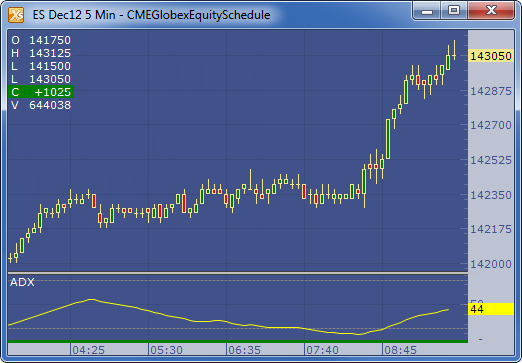← Back to X_TRADER® Help Library

### X_STUDY® Documentation

##### Description

The Average Directional Movement Index (ADX) is designed to quantify trend strength by measuring the amount of price movement in a single direction. The ADX is part of the Directional Movement system published by J. Welles Wilder, and is the average resulting from the Directional Movement indicators.

##### Formula

Directional Movement (DM) is defined as the largest part of the current period’s price range that lies outside the previous period’s price range. For each period calculate:

+DM =  positive or plus DM = High - Previous High

-DM = negative or minus DM = Previous Low - Low

The smaller of the two values is reset to zero, i.e., if +DM > -DM, then -DM = 0. On an inside bar (a lower high and higher low), both +DM and -DM are negative values, so both get reset to zero as there was no directional movement for that period.

The True Range (TR) is calculated for each period, where:

TR = Max of ( High - Low ), ( High -PreviousClose ), ( PreviousClose - Low )

The +DM, -DM and TR are each accumulated and smoothed using a custom smoothing method proposed by Wilder. For an n period smoothing, 1/n of each period’s value is added to the total each period, similar to an exponential smoothing:

+DMt = (+DMt-1 - (+DMt-1 / n))  + (+DMt)

-DMt = (-DMt-1 - (-DMt-1 / n)) + (-DMt)

TRt = (TRt-1 - (TRt-1 / n)) + (TRt)

Compute the positive/negative Directional Indexes, +DI and -DI, as a percentage of the True Range:

+DI = ( +DM / TR ) * 100

-DI = ( -DM / TR ) * 100

Compute the Directional Difference as the absolute value of the differences:   DIdiff = | ((+DI) - (-DI)) |

Sum the directional indicator values: DIsum = ((+DI) + (-DI)) .

Calculate the Directional Movement index:  DX = ( DIdiff / DIsum ) * 100 .  The DX is always between 0 and 100.

Finally, apply Wilder’s smoothing technique to produce the final ADX value:

ADXt = ( ( ADXt-1 * ( n - 1) ) + DXt ) / n

##### Example